Vectors - Motion and Forces in Two Dimensions - Lesson 3 - Forces in Two Dimensions

# Double Trouble in 2 Dimensions (a.k.a., Two Body Problems)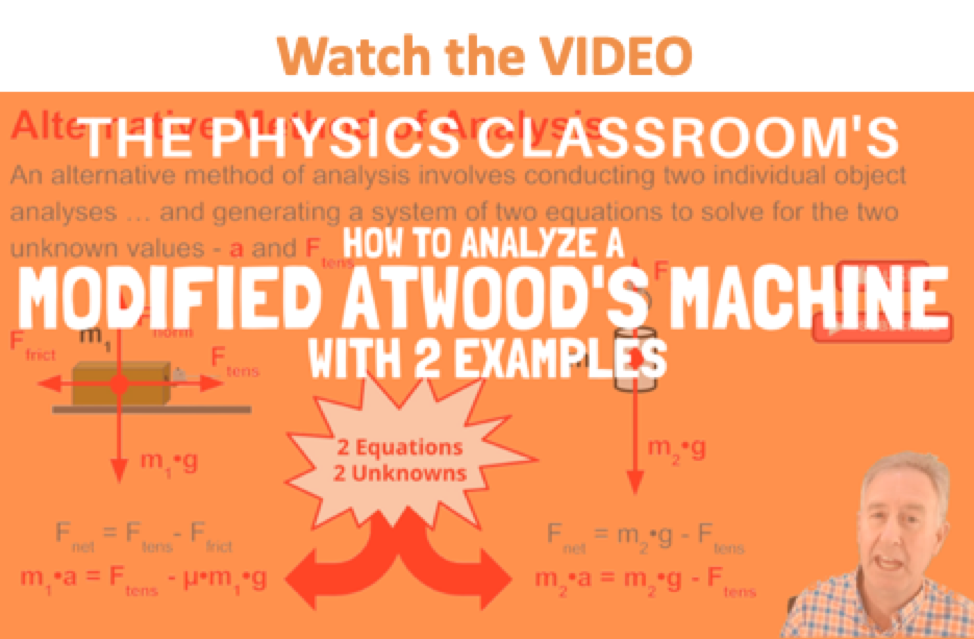In the Newton's laws unit, the topic of two-body problems was introduced. A pair of problem-solving strategies were discussed and applied to solve three example problems. Such two-body problems typically involve solving for the acceleration of the objects and the force that is acting between the objects. One strategy for solving two-body problems involves the use of a system analysis to determine the acceleration combined with an individual object analysis to determine the force transmitted between the objects. The second strategy involved the use of two individual object analyses in order to develop a system of two equations for solving for the two unknown quantities. If necessary, take the time to review the page on solving two-body problems. This page will build upon the lessons learned earlier in the Newton's Laws unit.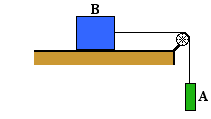In this lesson we will analyze two-body problems in which the objects are moving in different directions. In these problems, the two objects are connected by a string that transmits the force of one object to the other object. The string is wrapped around a pulley that changes the direction that the force is exerted without changing the magnitude. As an illustration of how a pulley works, consider the diagram at the right. Object A is connected to object B by a string. The string is wrapped around a pulley at the end of a table. Object A is suspended in mid-air while object B is on the table. In this situation, Object A will fall downward under the influence of gravity, pulling downward on one end of the string that it is connected to. According to Newton's law of action-reaction, this lower end of the string will pull upward on object A. The opposite end of the string is connected to object B. This end of the string pulls rightward upon object B. As such, the string connecting the two objects is pulling on both objects with the same amount of force, but in different directions. The string pulls upward on object A and rightward on object B. The pulley has changed the direction that the force is exerted.

Problems involving two objects, connecting strings and pulleys are characterized by objects that are moving (or even accelerating) in different directions. They move or accelerate at the same rate but in different directions. As such, it becomes important in approaching such problems to select a different reference frame and axes system for each object. Attention should be given to selecting an axes system such that both objects are accelerating along an axis in the positive direction. With the axes properly defined for each individual object, a free-body diagram can be constructed. Then Newton's laws can be applied to each diagram to develop a system of two equations for solving for the two unknowns. This problem-solving process will be demonstrated for three different example problems.

### Example Problem 1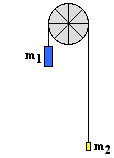A 200.0-gram mass (m1) and 50.0-gram mass (m2) are connected by a string. The string is stretched over a pulley. Determine the acceleration of the masses and the tension in the string.

As is frequently the case, this example problem requests information about two unknowns - the acceleration of the objects and the force acting between the objects. In a situation such as this one with two objects suspended over a pulley, the more massive object will accelerate downward and the least massive object will accelerate upward. The magnitude of the acceleration will be the same for each object. The coordinate system chosen for m1 has the positive y-axis directed downwards; the coordinate system chosen for m2 has the positive y-axis directed upwards. With this selection of axes, the direction of acceleration will be positive for each object. The free-body diagrams for each individual mass are shown below. Each object is experiencing a downward force of gravity - calculated as m1•g and m2•g respectively. Each object is also experiencing an upward tension force that pulls the two objects towards each other.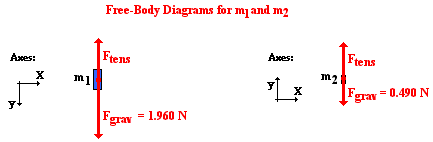Newton's second law equation (Fnet = m•a) can be applied to both diagrams in order to write two equations for the two unknowns. The Fnet will be expressed as the force in the direction of the acceleration minus the one that opposes it. So for the 200.0-gram mass, Fnet is written as 1.960 N - Ftens. For the 50.0-gram mass, Fnet is written as Ftens - 0.490 N. Equations 1 and 2 are the result of applying the Newton's second law equation to the 200.0-gram and 50.0-gram masses. (Note that the mass values are converted to the standard kilogram unit before use in the equations. Also note that the units have been dropped in order for the equations to read more cleanly.)

1.960 - Ftens = 0.2000•a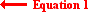Ftens - 0.490 = 0.0500•aFrom this point, a few steps of algebra lead to the answers to the problem. Equation 2 can be rearranged to develop an expression for Ftens written in terms of the acceleration.

Ftens = 0.0500•a + 0.490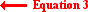This expression for Ftens can now be substituted into Equation 1 in order to change it into a single-unknown equation. That equation and the subsequent steps of algebra leading to the value of acceleration are shown below.

1.96 - (0.0500•a + 0.490) = 0.2000•a
1.96 - 0.0500•a - 0.490 = 0.2000•a
1.47 = 0.2500•a
a = 1.47/.2500 = 5.88 m/s2

Now that the acceleration has been found from Equation 1, its value can be substituted into Equation 3 in order to determine the tension.

Ftens = 0.0500•(5.88) + 0.490
Ftens = 0.784 N

The pulley system analyzed here is sometimes referred to as an Atwood's machine. The problem-solving approach is the standard approach that will be used throughout this page in order to solve for the two unknowns. It will be repeated in Example Problem 2 in order to solve what is commonly referred to as a modified Atwood's machine problem.

### Example Problem 2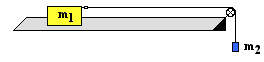Consider the two-body situation at the right. A 20.0-gram hanging mass (m2) is attached to a 250.0-gram air track glider (m1). Determine the acceleration of the system and the tension in the string.

As in Example Problem 1, this system must first be analyzed conceptually in order to determine the direction of acceleration of the two objects. This will allow for the assignment of a coordinate axes for each object. Since there is nothing pushing m1 to the left, we would reason that it would accelerate to the right due to the pull of the string. The hanging mass (m2) will clearly accelerate downward under the influence of gravity. Thus, the coordinate system is chosen for m2 has the positive y-axis directed downward; the coordinate system chosen for m1 has the positive x-axis directed rightward. With this selection of axes, the direction of acceleration will be positive for each object.

The free-body diagram for each individual mass is shown below. Each object is experiencing a downward force of gravity (Fgrav) - calculated as m1•g and m2•g respectively. The glider (m1) is experiencing an upward support force (air pushing up on it) to balance the force of gravity. The glider is also experiencing a horizontal force - the tension force (Ftens) to the right. The hanging mass (m2) is experiencing an upward tension force (Ftens) that offers some resistance to the downward pull of gravity.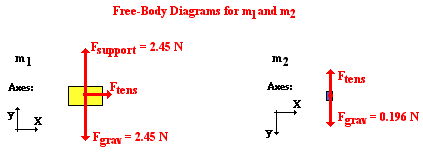Newton's second law equation (Fnet = m•a) can be applied to both free-body diagrams in order to write two equations for the two unknowns. The Fnet will be expressed as the force in the direction of the acceleration minus any that oppose it. For the 250.0-gram (0.250 kg) glider, Fnet is simply the unbalanced tension force (Ftens). For the 20.0-gram (0.020 kg) hanging mass, Fnet is written as 0.196 N - Ftens. Equations 4 and 5 are the result of applying the Newton's second law equation to the 250.0-gram glider and 20.0-gram hanging mass. (Note that the mass values are converted to the standard kilogram unit before use in the equations. Also note that the units have been dropped in order for the equations to read more cleanly.)

Ftens = 0.2500•a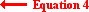0.196 - Ftens = 0.0200•a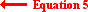From this point, a few steps of algebra lead to the answers to the problem. Equation 4 expresses the Ftens value in terms of the acceleration. This expression for Ftens can be substituted into Equation 5 in order to convert it to a single-unknown equation. That equation and the subsequent steps of algebra leading to the value of acceleration are shown below.

0.196 - 0.2500•a = 0.0200•a
0.196 = 0.2700•a
a = .196/.2700 = 0.72593 m/s2
a = ~0.726 m/s2

Now that the acceleration has been found from Equation 5, its value can be substituted into Equation 4 in order to determine the tension.

Ftens = 0.2500•(0.72593) = 0.18148
Ftens = ~0.181 N

The pulley system analyzed in Example Problem 2 is sometimes referred to as a modified Atwood's machine. The analysis is slightly more complicated than the Atwood's machine of Example Problem 1. The final example problem will be a case of a modified Atwood's machine with the surface inclined as shown below. The problem-solving approach will be the same.### Example Problem 3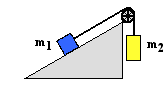Consider the two-body situation at the right. A 2.50x103-kg crate (m1) rests on an inclined plane and is connected by a cable to a 4.00x103-kg mass (m2). This second mass (m2) is suspended over a pulley. The incline angle is 30.0° and the surface is frictionless. Determine the acceleration of the system and the tension in the cable.

Like the previous problem, the first task involves analyzing the situation to determine which direction the objects will accelerate. Such an analysis will allow a coordinate axes system to be assigned to each object. In this case, the hanging mass (m2) could be accelerated upward or downward. The direction it accelerates depends upon a comparison of its weight (the force of gravity) to the opposing force acting on the other mass (m1). The mass on the inclined plane encounters three forces - the gravity force, the normal force and the tension force. The gravity force is directed downward (as is usual) and calculated as m1•g. The normal force is directed perpendicular to the surface (as is usual). The tension force is directed upwards and rightwards - parallel to the inclined plane and along the same orientation as the string that provides this force. As discussed on the previous page, objects placed on inclined planes are analyzed by resolving the force of gravity into two components. One component is directed parallel to the plane (and downwards at this angle) and the other component is directed perpendicular to the plane (and upwards at this angle). It is the parallel component of gravity that attempts to pull m1 down the inclined plane. As mentioned previously, this component can be calculated by multiplying the weight of the object (m1•g) by the sine of the incline angle (30°). The value for Fparallel is

Fparallel = m1•g•sine(θ) = (2500 kg)•(9.8 N/kg)•sine(30°)
Fparallel = 12250 N

This parallel component of gravity is attempting to pull m1 down the inclined plane. Since m1 is attached by the cable to m2, the hanging mass would be pulled with it. However, there is the opposing action of gravity pulling m2 downward; this opposing action, if dominant, would drag the object m1 up the inclined plane. The force of gravity on m2 is

Fgrav-2 = m2•g = (4000 kg)•(9.8 N/kg) = 39200 N

This gravitational force on m2 is the dominant force. And so m1 will accelerate up the inclined plane and m2 will accelerate downward. The coordinate axes are assigned accordingly so that each object has a positive acceleration.

The diagrams below show these coordinate axes and the forces acting upon the two objects. The three forces on m1 have already been discussed. The diagram shows the two components of Fgrav. As mentioned on the previous page, the perpendicular component of gravity is calculated as

Fperpendicular = m1•g•cosθ = (2500 kg)•(9.8 N/kg)•cos(30°)
Fperpendicular = 21218 N

The normal force (Fnorm) acting upon m1 balances the Fperpendicular so that there is no acceleration perpendicular to the inclined plane. The Fnorm value is also 21218 N. The hanging mass (m2) experiences only two forces - the downward pull of gravity and the upward tension force.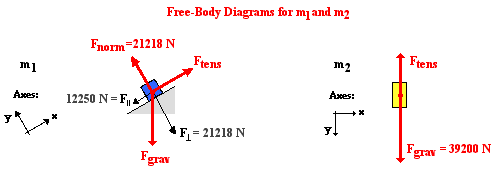Now the Newton's second law equation (Fnet = m•a) can be applied to both free-body diagrams in order to write two equations for the two unknowns. The Fnet is expressed as the force in the direction of the acceleration minus any that oppose it. For the 2500-kg mass on the incline (m1), Fnet is simply the tension force (Ftens) minus the parallel component of gravity. For the 4000-kg hanging mass (m2), Fnet is the force of gravity (39200 N) minus the tension force (Ftens). Equations 6 and 7 are the result of applying the Newton's second law equation to m1 and m2. (Note that the units have been dropped in order for the equations to read more cleanly.)

Ftens - 12250 = 2500•a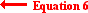39200 - Ftens = 4000•a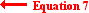From this point, a few steps of algebra lead to the answers to the problem. Equation 6 can be rearranged to create an expression for Ftens, expressed in terms of the acceleration.

Ftens = 2500•a + 12250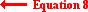This expression for Ftens can be substituted into Equation 7 in order to convert it to a single-unknown equation. That equation and the subsequent steps of algebra leading to the value of acceleration are shown below.

39200 - (2500•a + 12250) = 4000•a
39200 - 2500•a - 12250 = 4000•a
26950= 6500•a
a = 26950/6500 = 4.1462 m/s2
a = ~4.15 m/s2

Now that the acceleration has been found from Equation 7, its value can be substituted into Equation 8 in order to determine the tension force (Ftens).

Ftens = 2500•a + 12250 = 2500•(4.1462) + 12250 = 22615 N
Ftens = ~2.26 x 104 N

Two-body problems like these three example problems can be quite a challenge. Having a systematic approach that is applied to every problem simplifies the analysis. Good conceptual understanding, a commitment to the use of free-body diagrams, and a solid grasp of Newton's second law are the essential ingredients of success.

### We Would Like to Suggest ...Sometimes it isn't enough to just read about it. You have to interact with it! And that's exactly what you do when you use one of The Physics Classroom's Interactives. We would like to suggest that you combine the reading of this page with the use of our Atwood's Machine Simulator. You can find it in the Physics Interactives section of our website. The simulator allows one to explore two-mass systems accelerated by a hanging mass.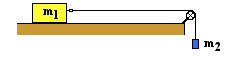1. Consider the two-body situation at the right. A 100.0-gram hanging mass (m2) is attached to a 325.0-gram mass (m1) at rest on the table. The coefficient of friction between the 325.0-gram mass and the table is 0.215. Determine the acceleration of the system and the tension in the string.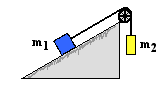2. Consider the two-body situation at the right. A 3.50x103-kg crate (m1) rests on an inclined plane and is connected by a cable to a 1.00x103-kg mass (m2). This second mass (m2) is suspended over a pulley. The incline angle is 30.0° and the surface has a coefficient of friction of 0.210. Determine the acceleration of the system and the tension in the cable.